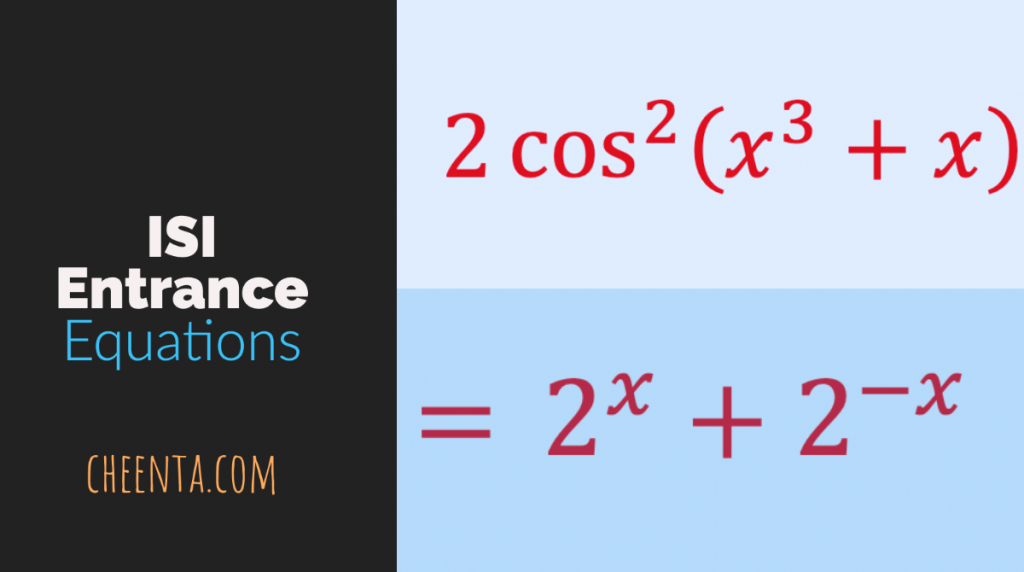How Cheenta works to ensure student success?
Explore the Back-Story

# Solving Weird Equations using Inequality | TOMATO Problem 78Here is a video solution for ISI Entrance Number Theory Problems based on solving weird equations using Inequality. Watch and Learn!

Here goes the question…

Solve: 2 \cos ^{2}\left(x^{3}+x\right)=2^{x}+2^{-x}

We will recommend you to try the problem yourself.

Done?

Let’s see the proof in the video below:

## Related Program

Here is a video solution for ISI Entrance Number Theory Problems based on solving weird equations using Inequality. Watch and Learn!

Here goes the question…

Solve: 2 \cos ^{2}\left(x^{3}+x\right)=2^{x}+2^{-x}

We will recommend you to try the problem yourself.

Done?

Let’s see the proof in the video below:

## Related Program

This site uses Akismet to reduce spam. Learn how your comment data is processed.

### Knowledge Partner